Calculate Latency and Doppler in a Satellite Scenario

This example shows how to model a satellite in orbit, analyze access between the satellite and a ground station, and calculate the latency and the doppler frequency between the satellite and the ground station.

Create a Satellite Scenario

Create a satellite scenario with a start time of 02-June-2020 8:23:00 AM UTC and a stop time 24 hours later. Set the simulation sample time to 60 seconds.

startTime = datetime(2020,6,02,8,23,0);
stopTime = startTime + hours(24);
sampleTime = 60;  % s
sc = satelliteScenario(startTime,stopTime,sampleTime);

Add a Satellite to the Scenario

Add a satellite to the scenario from the oneSatelliteConstellation TLE file.

sat = satellite(sc,"oneSatelliteConstellation.tle");

Show the satellite in orbit and plot its future ground track, or lead time, over 20 minutes. Purple shows the future ground track, and orange shows the past ground track.

show(sat)
groundTrack(sat, ...

Return Orbital Elements and Position of the Satellite

Display the orbital elements of the satellite in the scenario.

elements = orbitalElements(sat)
elements = struct with fields:
MeanMotion: 9.1649e-04
Eccentricity: 1.0000e-03
Inclination: 55
RightAscensionOfAscendingNode: 175.0000
ArgumentOfPeriapsis: 100
MeanAnomaly: 174.9900
Period: 6.8557e+03
Epoch: 02-Jun-2020 18:43:16
BStar: 1.0000e-04

Return the latitude (degrees), longitude (degrees), and altitude (km) of the satellite at time 02-June-2020 12:30:00 PM UTC.

time = datetime(2020,6,02,12,30,0);
pos = states(sat,time,"CoordinateFrame","geographic");
pos(3) = pos(3) / 1000;  % convert from m to km
fprintf("The satellite latitude is %3.2f degrees, its longitude is %5.2f degrees, and its altitude is %5.2f km.", ...
pos(1),pos(2),pos(3))
The satellite latitude is 1.07 degrees, its longitude is -83.95 degrees, and its altitude is 1421.20 km.

Add the Madrid Deep Space Communications Complex as the ground station of interest, and specify its latitude and longitude.

name = "Madrid Deep Space Communications Complex";
lat = 40.43139;  % degrees
lon = -4.24806;  % degrees
gs = groundStation(sc,"Name",name,"Latitude",lat,"Longitude",lon);

Add an access analysis between the satellite and the ground station, which determines when the ground station is visible to the satellite. This determines when latency and doppler should be calculated.

ac = access(sat,gs);
acStatus = accessStatus(ac);

Calculate Latency and Velocity

Calculate the latency between the satellite and the Madrid ground station. Also, calculate the velocity along the line between the satellite and the ground station. A positive value indicates that the satellite is moving towards the ground station, and a negative value indicates the satellite is moving away from the ground station.

numHours = stopTime - startTime;
numMinutes = minutes(numHours);
[az,el,r] = deal(zeros(numMinutes+1,1));
[satV,dir] = deal(zeros(3,numMinutes+1));
[latency,dopV] = deal(NaN(numMinutes+1,1));
c = physconst("Lightspeed");
for iMinute = 0:numMinutes
time = startTime + minutes(iMinute);
idx = iMinute+1;
% Calculate latency and doppler only when the satellite has access to the
% ground station.
if acStatus(idx)
% Calculate azimuth, elevation, and range from the satellite to the
% ground station
[az(idx),el(idx),r(idx)] = aer(sat,gs,time);

% Calculate latency
latency(idx) = r(idx) / c;

% Calculate satellite velocity in North/East/Down (NED) frame of
% satellite. Physically, this is the ECEF velocity, represented in NED
% frame. Therefore, the relative velocity with respect to the ground
% station is also the same.
[~,satV(:,idx)] = states(sat,time,"CoordinateFrame","geographic");

% Calculate the direction of gs with respect to sat in sat NED frame
dir(:,idx) = [cosd(el(idx))*cosd(az(idx)); ...
cosd(el(idx))*sind(az(idx)); ...
-sind(el(idx))];

% Calculate the velocity along the line between the gs and the sat.
% This velocity determines the doppler frequency.
dopV(idx) = dot(satV(:,idx),dir(:,idx));
end
end
plot(1000*latency)  % ms
title("Latency vs. Time")
xlabel("Simulation Time (s)")
ylabel("Latency (ms)")
grid on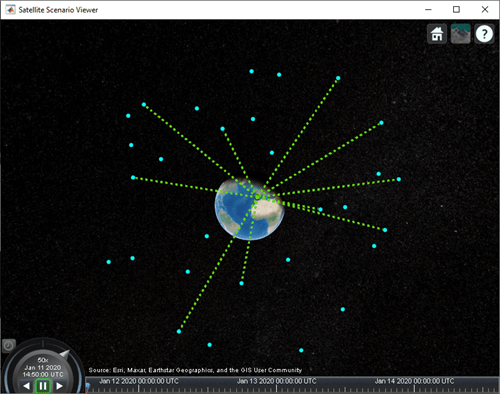plot(dopV)
title("Satellite Velocity Along the Line Between Sat and GS")
xlabel("Simulation Time (s)")
ylabel("Velocity (m/s)")
grid on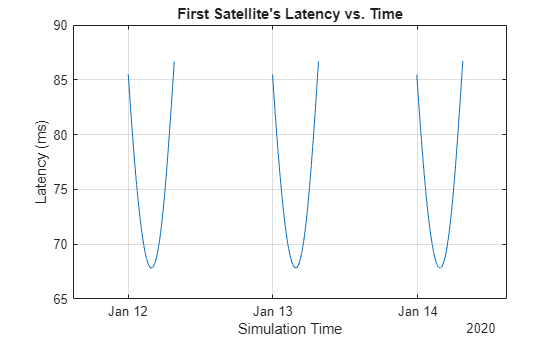Calculate Doppler Frequency from Velocity

The doppler frequency is calculated from the following equation:

${\mathit{f}}_{\mathit{o}}=\left(\frac{\mathit{c}±{\mathit{v}}_{\mathit{r}}}{\mathit{c}±{\mathit{v}}_{\mathit{s}}}\right){\mathit{f}}_{\mathit{e}}$,

where $\mathit{c}$ is the speed of light in m/s,

${\mathit{v}}_{\mathit{r}}$ is the speed, in m/s, of the receiver relative to the medium, added to $\mathit{c}$ if the receiver is moving towards the source, subtracted if the receiver is moving away from the source,

${\mathit{v}}_{\mathit{s}}$ is the speed, in m/s, of the source relative to the medium, added to $\mathit{c}$ if the source is moving away from the receiver, subtracted if the source is moving towards the receiver,

${\mathit{f}}_{\mathit{e}}$ is the emitted frequency, in Hz, and

${\mathit{f}}_{\mathit{o}}$ is the observed frequency, in Hz.

In this example, we consider the observed frequency from the standpoint of a receiving ground station. In that case, ${\mathit{v}}_{\mathit{r}}$ is 0. We also consider a C band frequency of 5 GHz.

fe = 5e9;  % emitted frequency in Hz
fo = (((c ./ (c-dopV)) * fe) - 5e9);  % doppler shift in Hz
plot(fo/1e3)  % plot kHz
title("Doppler Shift vs. Time")
xlabel("Simulation Time (s)")
ylabel("Doppler Shift (kHz)")
grid onCalculate Rates of Change for Latency and Doppler

Satellite communications links need to be designed to track varying latencies and Doppler frequencies. Thus, it is important to calculate these quantities.

latencyRate = diff(latency)/sampleTime;
plot(1000*latencyRate)  % ms/s
title("Latency Rate of Change")
xlabel("Simulation Time (s)")
ylabel("Latency Rate of Change (ms/s)")
grid on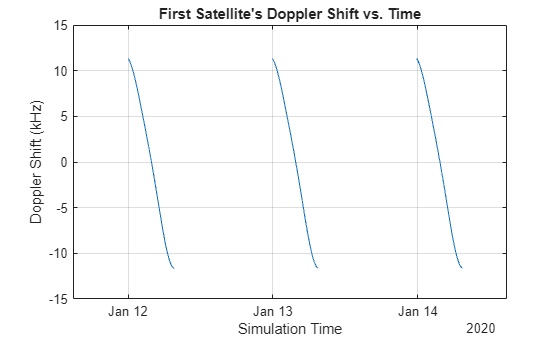dopplerRate = (diff(fo)/sampleTime);
plot(dopplerRate)
title("Doppler Rate of Change")
xlabel("Simulation Time (s)")
ylabel("Doppler Rate of Change (Hz/s)")
grid on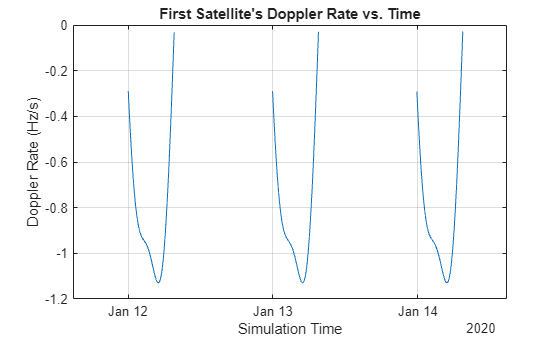Play the scenario with the satellite and ground station.

play(sc)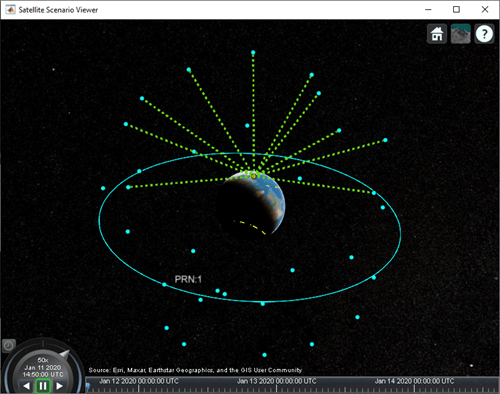Related Examples